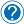How to parse an array in php

I remember back when I first started programming that I was having a bit of hard time when parsing an array in any giving language (well only php or java at the time), its always like that, after you learn it, you always think its very simple. Well same is for me, I’m not trying to brag, but I will show 3 methods of parsing an array really quick in PHP. The logic is similar in any programming language, be it php, perl, python, c, c++ etc., the logic is the same, but the syntax may be slightly different. So yeah, straight to the point, how to parse an array in php.

1. Loop through array using foreach

This is the simplest method there is and its also really fast. I’ll give you two examples for two arrays, one is a normal array and one is associative array.

The above will return each element at a time on each line. Now for associative arrays, this is almost the same.

This will print key1 – elem_1 on each line. And that is foreach method to loop through the array.

2. Loop through array using for

Using this method is a bit different, this works by iterating through the array keys. The logic here is that you declare a variable that is equal to zero (the first array key) and then you increment the variable by one on each iteration until you reach the total number of elements in the array. An example is bellow:

The above is similar to the first foreach method, it will print each element on each line.

3. Loop through array using while

This method is similar to the previous one, for, has the same principle to increment a variable until you reach the total number of elements from the array. The associative array part though is a bit different. Bellow you have the examples:

So its really similar to the previous method, now the associative array, as I said, it is slightly different:

This will print the output similar to the first method, foreach, key1 – elem_1. And that is how you parse any given array. Now the method selected doesn’t really matter, but foreach and while appear to be used the most as I’ve seen.Request an article ←